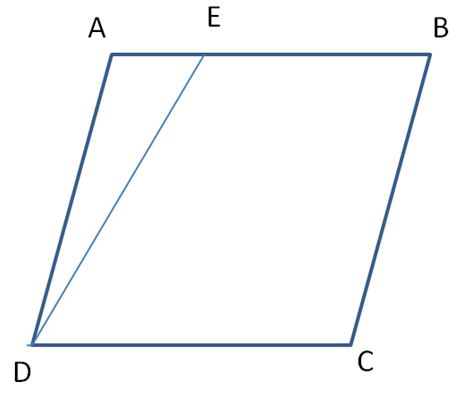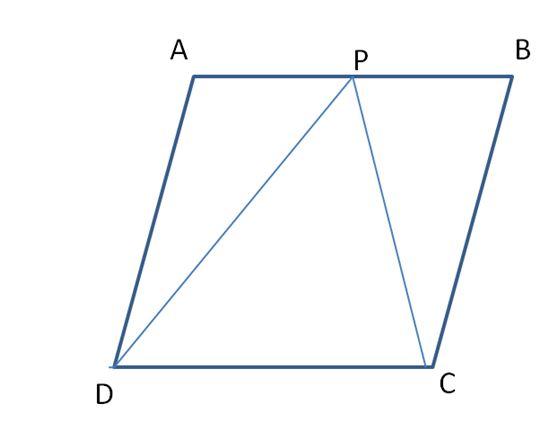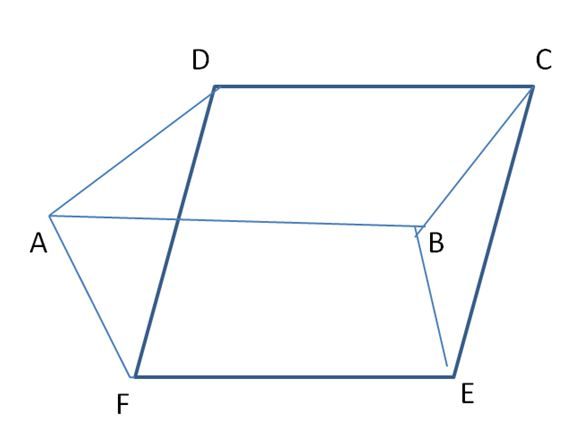Students can check how parallelograms having the same base and between the same parallels are equal in area and proof for that. When two or more parallelogram lies on the same base and between same parallels then they have the same area. Also, find the solved example questions here explaining the concept and learn the concept behind them.

## Proof for Theorem: Parallelogram on the Same Base & Between Same Parallels are Equal in Area

Let us draw a parallelogram ABCD on a cardboard sheet or thick sheet of paper.Now, draw a line segment DE as shown in the figure.Now, cut the triangle ADE congruent to triangle ADE in a seperate sheet with the help of a tracing paper and place the triangle ADE in such a way that AD coincides BC. The entire process is as shown below.Note that there are two parallelograms ABCD and EE’CD on the same base CD and between the same parallel AE’ and CD.

∆ADE ≅ ∆ A’ D’ E’

Therefore, Area of ADE = Area of A’D’E’

Also, Area of ABCD = Area of ADE + Area of EBCE

= Area of A’D’E’ + Area of EBCD

= Area of EE’CD

So, the two parallelograms are in an equal area.

### Parallelograms on Same Base and Between Same Parallels Examples

Example 1:

A farmer was having a field in the form of a parallelogram ABCD. She took any point P on AB and joined it to points C and D. In how many parts the fields are divided? What are the shapes of these parts? The farmer wants to sow wheat and pulses in equal portions of the field separately. How should she do it?Solution:

If DP and CP are joined parallelogram ABCD is divided into three triangles.

They are △ APD, △ CDP, △ BCP

△ CDP and parallelogram ABCD are on the same base i.e CD and between parallel sides CD ∥ AC

∴ Area of △ CDP = 1/2 x area of parallelogram ABCD —- (i)

∴ Area of △ APD + Area of △ BCP = 1/2 x area of parallelogram ABCD —- (ii)

From equation (i) and equation (ii)

Area of △ CDP = Area of △ APD + Area of △ BCP

∴ The farmer can use the part of the field to sow wheat, i.e. Area of △ APD + Area of △ BCP, and in the same area he can use the Area of △ CDP to sow pulses.

Example 2:

Parallelograms ABCD and ABEF are situated on the opposite sides of AB in such a way that D, A, F are not collinear. Prove that DCEF is a parallelogram, and parallelogram ABCD + parallelogram ABEF = parallelogram DCEF.

Solution:AB and DC are two opposite sides of parallelogram ABCD,

Therefore, AB ∥ DC and AB = DC

Again, AB and EF are two opposite sides of parallelogram ABEF

Therefore, AB ∥ EF and AB ∥ EF

Therefore, DC ∥ EF and DC = EF

Therefore, DCEF is a parallelogram.

Therefore, ∆ADF and ∆BCE, we get

AD = BC (opposite sides of parallelogram ABCD)

AF = BE (opposite sides of parallelogram ABEF)

And DF = CE (opposite sides of parallelogram CDEF)

Therefore, ∆ADF ≅ ∆BCE (side – side – side)